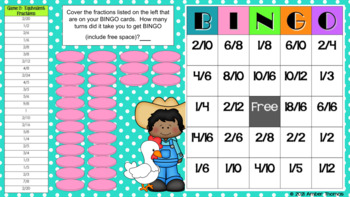DID YOU KNOW:
Seamlessly assign resources as digital activities

Learn how in 5 minutes with a tutorial resource. Try it Now

Learn More# Math Fractions Games for Kids: BingoGrade Levels
3rd - 4th, Homeschool
Subjects
Standards
Resource Type
Formats Included
• PDF
Pages
12+

#### Also included in

1. This product contains 3 fun fraction activities to help your students practice finding equivalent fractions. This bundle is more economical than buying each of these activities separately. The activities included are:❶ Fraction Bingo❷ Chocolate Fractions❸ Trading FractionsThese activities are in W
\$4.99
\$7.00
Save \$2.01

### Description

Are you looking for math fractions games that you can play with the whole class or a small group? Try Fraction Bingo! You can reinforce different math concepts depending on which "answer guide" you read from. So whether you are looking for adding fractions games, equivalent fractions games, or simply identifying fractions games, this product will meet your needs.

Update: This product now includes digital activities. The .pdf contains a link to the Google Slide version.

This product includes:
✅ One sheet that contains 4 different Bingo cards (all 4 cards can be used for all 3 games)
✅ A teacher answer sheet for the 3 different games, depending on your objectives
✅ Instructions on how to set up your game

You will need:
► Markers such as coins, torn paper, or beans for each child to cover his/her answers
► Card stock to copy the Bingo cards onto
► Scissors to cut out the cards

So whether you are looking for 3rd or 4th grade fraction games, I think you'll find Bingo an easy game to set up, play yourself, or leave for a substitute teacher.

✨✨✨Are you looking for more ways to help your students deeply understand fractions? I have a whole range of fractions items here! Check out these popular products✨✨✨

Chocolate Fractions: Equivalent Fractions Activity
Common Fractions and Improper Fractions Font
Equivalent Fractions Activities 3 in One Bundle
Equivalent Fractions Game: Trading Fractions
Fraction Circles Clipart {Commercial Use}
Fraction Line Plot Bundle
Fraction Line Plot Task Cards {Differentiated}
Fraction Line Plot Worksheets
Fraction Line Plots: Peer Surveys Open Ended Project
Fraction Number Line Game: Long Jump
Fraction Number Line Worksheets
Fraction of a Set Task Cards Bundle of 3
Fractions Font and Fraction Circle Graphics {Value Pack}
Fractions Unit for Fourth Grade
Line Up! Compare and Order Fractions
Using One Half as a Benchmark Fraction: Coloring Page

Total Pages
12+
Answer Key
Included
Teaching Duration
30 minutes
Report this Resource to TpT
Reported resources will be reviewed by our team. Report this resource to let us know if this resource violates TpT’s content guidelines.

### Standards

to see state-specific standards (only available in the US).
Compare two fractions with different numerators and different denominators, e.g., by creating common denominators or numerators, or by comparing to a benchmark fraction such as 1/2. Recognize that comparisons are valid only when the two fractions refer to the same whole. Record the results of comparisons with symbols >, =, or <, and justify the conclusions, e.g., by using a visual fraction model.
Explain why a fraction 𝘢/𝘣 is equivalent to a fraction (𝘯 × 𝘢)/(𝘯 × 𝘣) by using visual fraction models, with attention to how the number and size of the parts differ even though the two fractions themselves are the same size. Use this principle to recognize and generate equivalent fractions.
Recognize and generate simple equivalent fractions, (e.g., 1/2 = 2/4, 4/6 = 2/3). Explain why the fractions are equivalent, e.g., by using a visual fraction model.
Understand two fractions as equivalent (equal) if they are the same size, or the same point on a number line.
Explain equivalence of fractions in special cases, and compare fractions by reasoning about their size.

### Questions & Answers

Teachers Pay Teachers is an online marketplace where teachers buy and sell original educational materials.

More About Us